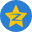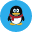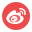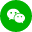# php使用循环计算1-2+3-4+5-6+…-100的值？

`计算1-2+3-4+5-6+…-100的值`，可以发现规律数字是依次递增的，而从第一位数后的运算符在偶数时是-，在奇数时是+。通过这个规律最终可以使用一次循环和对每次递增数字奇偶判断并运算最终得到结果。

php使用循环计算`1-2+3-4+5-6+…-100 = -50`

代码示例：

```<?php
\$sum = 0;
\$i = 1;
while (\$i <= 100) {
if (\$i == 1) {
\$sum = 1;
} else {
if (\$i % 2 == 0) {
\$sum -= \$i;
} else {
\$sum += \$i;
}
}
\$i++;
}

echo '1-2+3-4+5-6+…-100 = ' . \$sum;```

`1-2+3-4+5-6+…-100 = -50`IN: